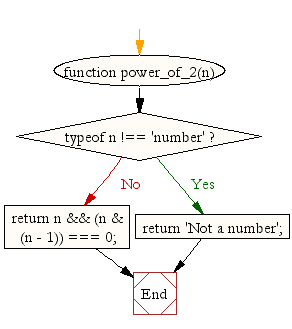# JavaScript: Test if a number is a power of 2

## JavaScript Math: Exercise-13 with Solution

Write a JavaScript function to test if a number is a power of 2.

Test Data:
console.log(power_of_2(16));
console.log(power_of_2(18));
console.log(power_of_2(256));
Output:
true
false
true

Pictorial Presentation:Sample Solution-1:

JavaScript Code:

``````function power_of_2(n) {
if (typeof n !== 'number')
return 'Not a number';

return n && (n & (n - 1)) === 0;
}

console.log(power_of_2(16));
console.log(power_of_2(18));
console.log(power_of_2(256));
```
```

Sample Output:

```true
false
true
```

Flowchart:Sample Solution-2:

JavaScript Code:

``````const power_of_2 = n => !!n && (n & (n - 1)) == 0;
console.log(power_of_2(16));
console.log(power_of_2(18));
console.log(power_of_2(256));
```
```

Sample Output:

```true
false
true
```

Live Demo:

See the Pen javascript-math-exercise-13 by w3resource (@w3resource) on CodePen.

Improve this sample solution and post your code through Disqus

What is the difficulty level of this exercise?

Test your Programming skills with w3resource's quiz.

﻿